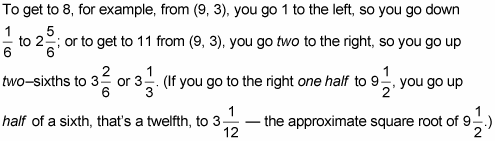##### Differential Equations For Dummies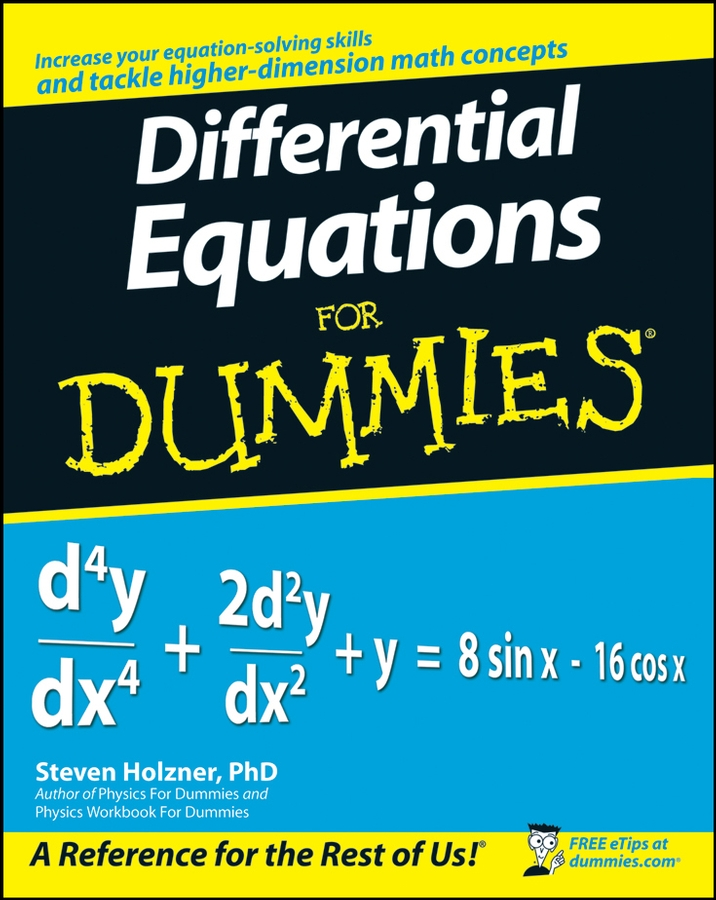Because ordinary functions are locally linear (that means straight) — and the further you zoom in on them, the straighter they look—a line tangent to a function is a good approximation of the function near the point of tangency.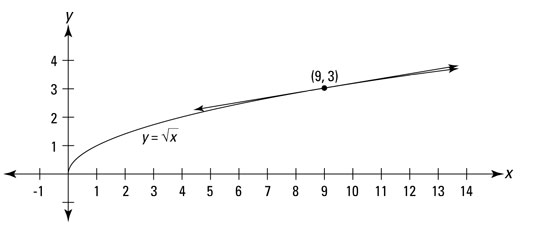This figure shows the graph of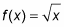and a line tangent to the function at the point (9, 3). You can see that near (9, 3), the curve and the tangent line are virtually indistinguishable.

Determining the equation of this tangent line is a breeze. You’ve got a point, (9, 3), and the slope is given by the derivative of f at 9: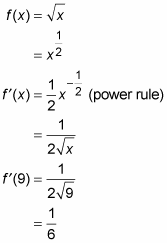Now just take this slope, 1/6, and the point (9, 3), and plug them into the point-slope form: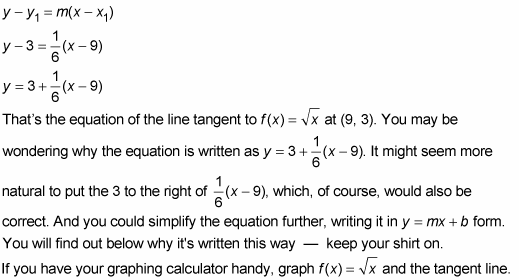Zoom in on the point (9, 3) a couple times, and you see that the curve gets straighter and straighter and the curve and tangent line get closer and closer.

Now, say you want to approximate the square root of 10. Because 10 is pretty close to 9, and because you can see from the figure that f(x) and its tangent line are close to each other at x = 10, the y-coordinate of the line at x = 10 is a good approximation of the function value at x = 10, namely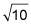Just plug 10 into the line equation for your approximation: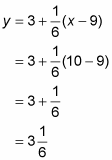Thus, the square root of 10 is about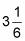This is only about 0.004 more than the exact answer of 3.1623….The error is roughly a tenth of a percent.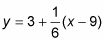This form makes it easier to do the computation and easier to understand what’s going on when you compute a linear approximation. Here’s why. You know that the tangent line goes through the point (9, 3), right? And you know the slope of the line is 1/6. So, you can start at (9, 3) and go to the right (or left) along the line in the stair-step fashion, as shown in the figure below: over 1, up 1/6; over 1, up 1/6, and so on.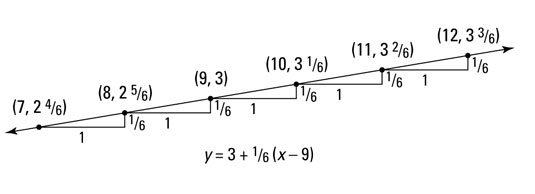So, when you’re doing an approximation, you start at a y-value of 3 and go up 1/6 for each 1 you go to the right. Or if you go to the left, you go down 1/6 for each 1 you go to the left. When the line equation is written in the above form, the computation of a linear approximation parallels this stair-step scheme.

The figure shows the approximate values for the square roots of 7, 8, 10, 11, and 12. Here’s how you come up with these values.